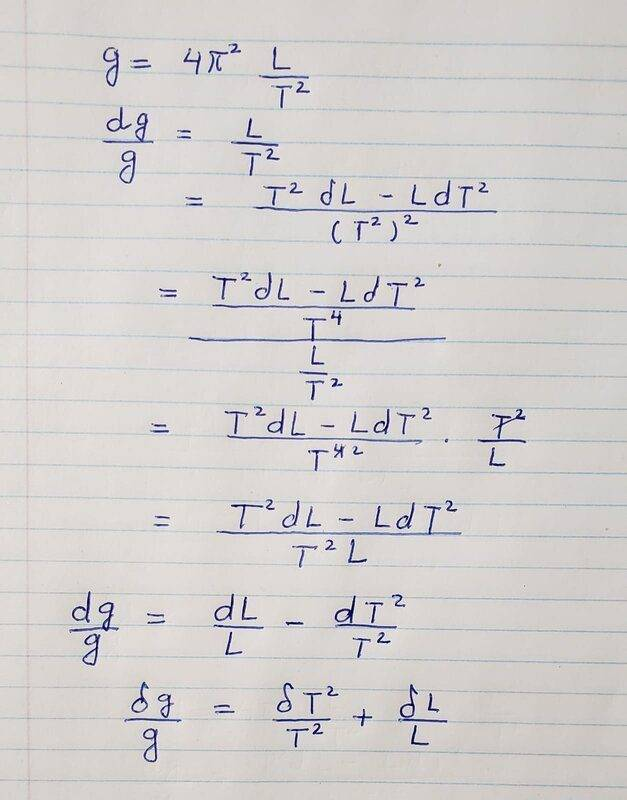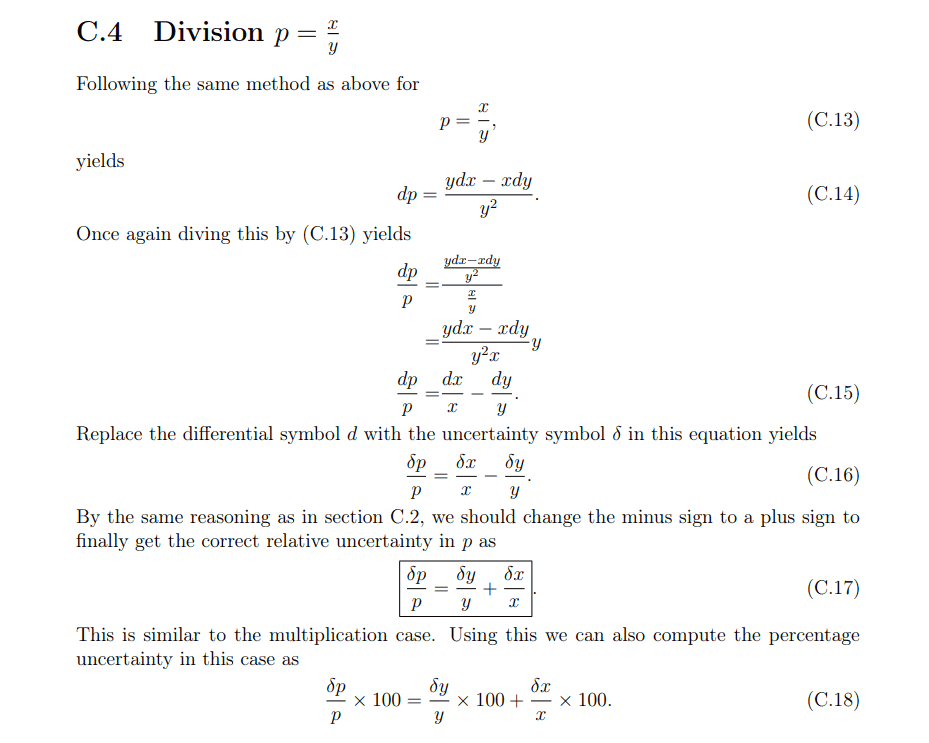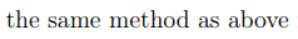# Finding the Uncertainty in the motion of a pendulum

• aatari
I will go back and try to understand what you are saying. In summary, the general formula for the (infinitesimal) variation in a function of two variables f(x,y) in terms of the (infinitesimal) variation in ##x## and ##y## is: $${df\over dx} = f'\ \Rightarrow \ df = f'\, dx$$ or (for small ##\Delta x##) : $$\Delta f \approx f'\,\Delta x$$f

#### aatari

Homework Statement
What is the expression for the uncertainty in finding from the motion of a pendulum
g = 4π^2 L/T^2

Assuming an uncertainty in L of δL, and uncertainty in T of δT.
Relevant Equations
g = 4π^2 L/T^2
Hi guys can someone look at my work for uncertainty and let me know if it makes sense.I don't even understand the first step. Where did you find that ?
Nor do I understand the last step. What are you doing there ?

What is the general formula for the (infinitesimal) variation in a function of two variables ##f(x,y)## in terms of the (infinitesimal) variation in ##x## and ##y## ?

Are you still there ?

sorry just seeing your message now. Actually the question asked to show the expression of uncertainty. I asked the TA and this is apparently correct based on the method in the attached image.What is the general formula for the (infinitesimal) variation in a function of two variables f(x,y) in terms of the (infinitesimal) variation in ##x## and ##y## ?
My hunch is that this question and yourrefer to one and the same:

For a function of one variable we write (casually) $${df\over dx} = f'\ \Rightarrow \ df = f'\, dx$$ or (for small ##\Delta x##) : $$\Delta f \approx f'\,\Delta x$$

In the case of two variables this becomes $$df = {\partial f\over \partial x} dx + {\partial f\over \partial y} dy$$leading to (C.14) in your image for the case ##f = {x\over y}##.

In your post #1, however, you write $$g = 4\pi^2\, {L\over T^2}$$$${dg\over g} = {L\over T^2}$$which is something else and simply wrong (effectively, it says ## g = 4\pi^2##)

Could it be you meant $${dg\over g} = { d\,{L\over T^2} \over {L\over T^2}}\quad ?$$

I suggest you go a step back to the general formula and work out ##\partial g\over \partial L## and ##\partial g\over \partial T## to come to an expression for ##dg##.

Once you fully understand that, we can work out an alternative approach using C.4 (and, as I suppose C.3).

•aatari
My hunch is that this question and your View attachment 272252 refer to one and the same:

For a function of one variable we write (casually) $${df\over dx} = f'\ \Rightarrow \ df = f'\, dx$$ or (for small ##\Delta x##) : $$\Delta f \approx f'\,\Delta x$$

In the case of two variables this becomes $$df = {\partial f\over \partial x} dx + {\partial f\over \partial y} dy$$leading to (C.14) in your image for the case ##f = {x\over y}##.

In your post #1, however, you write $$g = 4\pi^2\, {L\over T^2}$$$${dg\over g} = {L\over T^2}$$which is something else and simply wrong (effectively, it says ## g = 4\pi^2##)

Could it be you meant $${dg\over g} = { d\,{L\over T^2} \over {L\over T^2}}\quad ?$$

I suggest you go a step back to the general formula and work out ##\partial g\over \partial L## and ##\partial g\over \partial T## to come to an expression for ##dg##.

Once you fully understand that, we can work out an alternative approach using C.4 (and, as I suppose C.3).